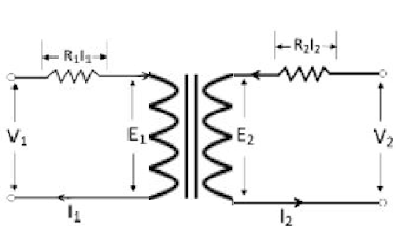WAZIPOINT Engineering Science & Technology: Transformer with Losses but no Magnetic Leakage

## How does loss without Magnetic Leakage in a Transformer?

To base our knowledge about the electrical transformer, we assumed an ideal transformer i.e. one in which there were no core losses and copper losses.

But in practical conditions of a real transformer, it requires that certain modifications be made in the foregoing theory.

When an actual transformer is put on the load, there is an iron loss in the transformer core and copper loss in the transformer windings both in primary and secondary coils and these losses are not entirely negligible.

Considering an actual transformer, there will be some resistance in both primary & secondary windings due to the presence of copper winding.Transformer with Losses but no Magnetic Leakage

Let us describe what is the effect in the transformer due to the presence of winding resistance but no magnetic leakage.

Let consider,
R1 = Primary winding resistance,
R2 =Secondary winding resistance,
V1= Primary applied voltage,
V2=Secondary terminal voltage,
E1= Primary induced e.m.f,
E2= Secondary induced e.m.f,
I1= Primary current,
I2= Secondary Current, ( which are shown in fig.)

Due to the presence of secondary winding resistance ( R2), an amount I2R2 voltage drop will occur in the secondary winding.
Hence, the secondary terminal voltage (V2) is equal to the vector difference of E2 and resistance voltage drop I2R2,

i.e E2=V2-I2R2

Similarly, Primary induced e.m.f E1 is equal to the vector difference of V1 and primary resistance voltage drop I1R1,

i.e E1=V1 - I1R1

We shall consider the following two cases:
1. When a transformer is on no-load;
2. When a transformer is on load.

A transformer is said to be on no-load if its secondary side is open and primary is connected to a sinusoidal alternating voltage V1 The alternating applied voltage will cause flow of alternating current in the primary winding which will create alternating flux.